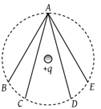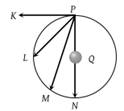The electric field inside a spherical shell of uniform surface charge density is -

(1) Zero

(2) Constant, less than zero

(3) Directly proportional to the distance from the centre

(4) None of the above

Concept Questions :-

Relation between field and potential
High Yielding Test Series + Question Bank - NEET 2020

Difficulty Level:

The electric potential V at any point O (x, y, z all in metres) in space is given by $V=4{x}^{2}\text{\hspace{0.17em}}volt$. The electric field at the point $\left(1m,\text{\hspace{0.17em}}0,\text{\hspace{0.17em}}2m\right)$ in volt/metre is -

(1) 8 along negative x-axis

(2) 8 along positive x-axis

(3) 16 along negative x-axis

(4) 16 along positive z-axis

Concept Questions :-

Relation between field and potential
High Yielding Test Series + Question Bank - NEET 2020

Difficulty Level:

A hollow metal sphere of radius 5 cm is charged so that the potential on its surface is 10 V. The potential at the centre of the sphere is -

(1) 0 V

(2) 10 V

(3) Same as at point 5 cm away from the surface

(4) Same as at point 25 cm away from the surface

Concept Questions :-

Electric potential
High Yielding Test Series + Question Bank - NEET 2020

Difficulty Level:

If a unit positive charge is taken from one point to another over an equipotential surface, then -

(1) Work is done on the charge

(2) Work is done by the charge

(3) Work done is constant

(4) No work is done

Concept Questions :-

Equipotential surfaces
High Yielding Test Series + Question Bank - NEET 2020

Difficulty Level:

In the electric field of a point charge q, a certain charge is carried from point A to B, C, D and E. Then the work done(1) Is least along the path AB

(2) Is least along the path AD

(3) Is zero along all the paths AB, AC, AD and AE

(4) Is least along AE

Concept Questions :-

Equipotential surfaces
High Yielding Test Series + Question Bank - NEET 2020

Difficulty Level:

A conductor with a positive charge

(1) Is always at +ve potential

(2) Is always at zero potential

(3) Is always at negative potential

(4) May be at +ve, zero or –ve potential

Concept Questions :-

Electric potential
High Yielding Test Series + Question Bank - NEET 2020

Difficulty Level:

Two spheres A and B of radius 4 cm and 6 cm are given charges of 80 μc and 40μc respectively. If they are connected by a fine wire, the amount of charge flowing from one to the other is -

(1) 20μc from A to B

(2) 16μc from A to B

(3) 32μc from B to A

(4) 32μc from A to B

Concept Questions :-

Electric potential
High Yielding Test Series + Question Bank - NEET 2020

Difficulty Level:

On rotating a point charge having a charge q around a charge Q in a circle of radius r. The work done will be

(1) $q×2\pi r$

(2) $\frac{q×2\pi Q}{r}$

(3) Zero

(4) $\frac{Q}{2{\epsilon }_{0}r}$

Concept Questions :-

Equipotential surfaces
High Yielding Test Series + Question Bank - NEET 2020

Difficulty Level:

Two charge +q and –q are situated at a certain distance. At the point exactly midway between them -

(1) Electric field and potential both are zero

(2) Electric field is zero but the potential is not zero

(3) Electric field is not zero but the potential is zero

(4) Neither electric field nor potential is zero

Concept Questions :-

Electric potential
High Yielding Test Series + Question Bank - NEET 2020

Difficulty Level:

In the figure the charge Q is at the centre of the circle. Work done is maximum when another charge is taken from point P to(1) K

(2) L

(3) M

(4) N

Concept Questions :-

Electric potential